# Parabolas

A quadratic function is a function that can be written in the form $f\left(x\right)=a{x}^{2}+bx+c$ where $a,b$, and $c$ are real numbers and $a\ne 0$. This form is called the standard form of a quadratic function.

The graph of the quadratic function is a U-shaped curve is called a parabola.

The graph of the equation $y={x}^{2}$, shown below, is a parabola. (Note that this is a quadratic function in standard form with $a=1$ and $b=c=0$.)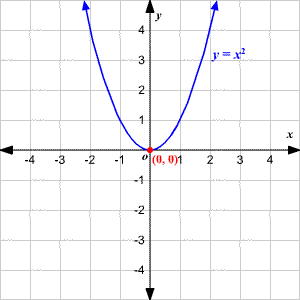In the graph, the highest or lowest point of a parabola is the vertex. The vertex of the graph of $y={x}^{2}$ is $\left(0,0\right)$.

If $a>0$ in $f\left(x\right)=a{x}^{2}+bx+c$, the parabola opens upward. In this case the vertex is the minimum, or lowest point, of the parabola. A large positive value of $a$ makes a narrow parabola; a positive value of $a$ which is close to $0$ makes the parabola wide.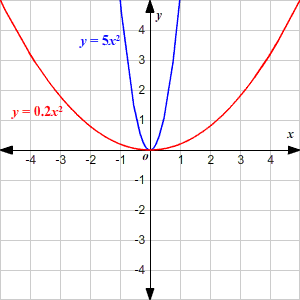If $a<0$ in $f\left(x\right)=a{x}^{2}+bx+c$, the parabola opens downward. In this case the vertex is the maximum, or highest point, of the parabola. Again, a large negative value of $a$ makes the parabola narrow; a value close to zero makes it wide.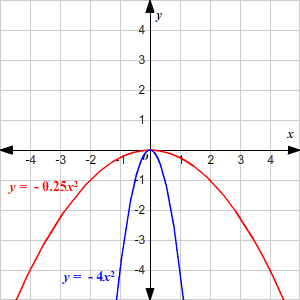For an equation in standard form, the value of $c$ gives the $y$-intercept of the graph.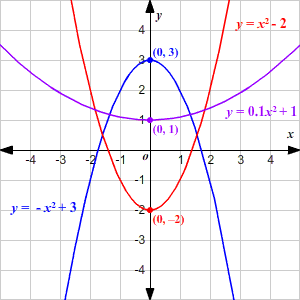The line that passes through the vertex and divides the parabola into two symmetric parts is called the axis of symmetry.

The equation of the axis of symmetry for the graph of $y=a{x}^{2}+bx+c$, where $a\ne 0$, is $x=-\frac{b}{2a}$

In all the above graphs, the axis of symmetry is the $y$-axis, $x=0$. In the graphs below, the axis of symmetry is different (marked in red.) Note that $c$ still gives the $y$-intercept.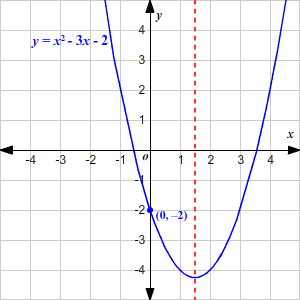If you write a quadratic function like $x=f\left(y\right)=a{y}^{2}+by+c$, where $x$ is a function of $y$ (instead of a $y$ as a function of $x$), you get a parabola where the axis of symmetry is horizontal.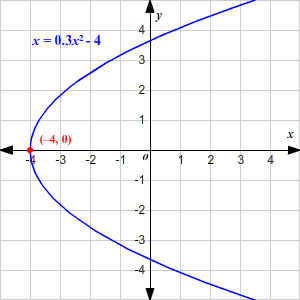Note that in this case, $c$ is the $x$-intercept. If $a$ is positive, the graph opens to the right; if $a$ is negative, the graph opens to the left.

Example:

Write the equation of the axis of symmetry, and find the coordinates of the vertex of the parabola $y=-3{x}^{2}-6x+4$.

The equation of the axis of symmetry for the graph of $y=a{x}^{2}+bx+c$.

$x=-\frac{b}{2a}$

Substitute $-3$ for $a$ and $-5$ for $b$ in the equation of the axis of symmetry.

$\begin{array}{l}x=-\frac{-6}{2\left(-3\right)}\\ \text{\hspace{0.17em}}\text{\hspace{0.17em}}\text{\hspace{0.17em}}\text{\hspace{0.17em}}=-1\end{array}$

So, the equation of the axis of symmetry is $x=-1$.

Since the equation of the axis of symmetry is $x=-1$ and the vertex lies on the axis, the $x$-coordinate of the vertex is $-1$.

To find the $y$-coordinate of the vertex, first substitute $-1$ for $x$ in the given equation.

$y=-3{\left(-1\right)}^{2}-6\left(-1\right)+4$

Simplify.

$\begin{array}{l}y=-3+6+4\\ \text{\hspace{0.17em}}\text{\hspace{0.17em}}\text{\hspace{0.17em}}\text{\hspace{0.17em}}=7\end{array}$

Therefore, the coordinates of the vertex of the parabola is $\left(-1,7\right)$.